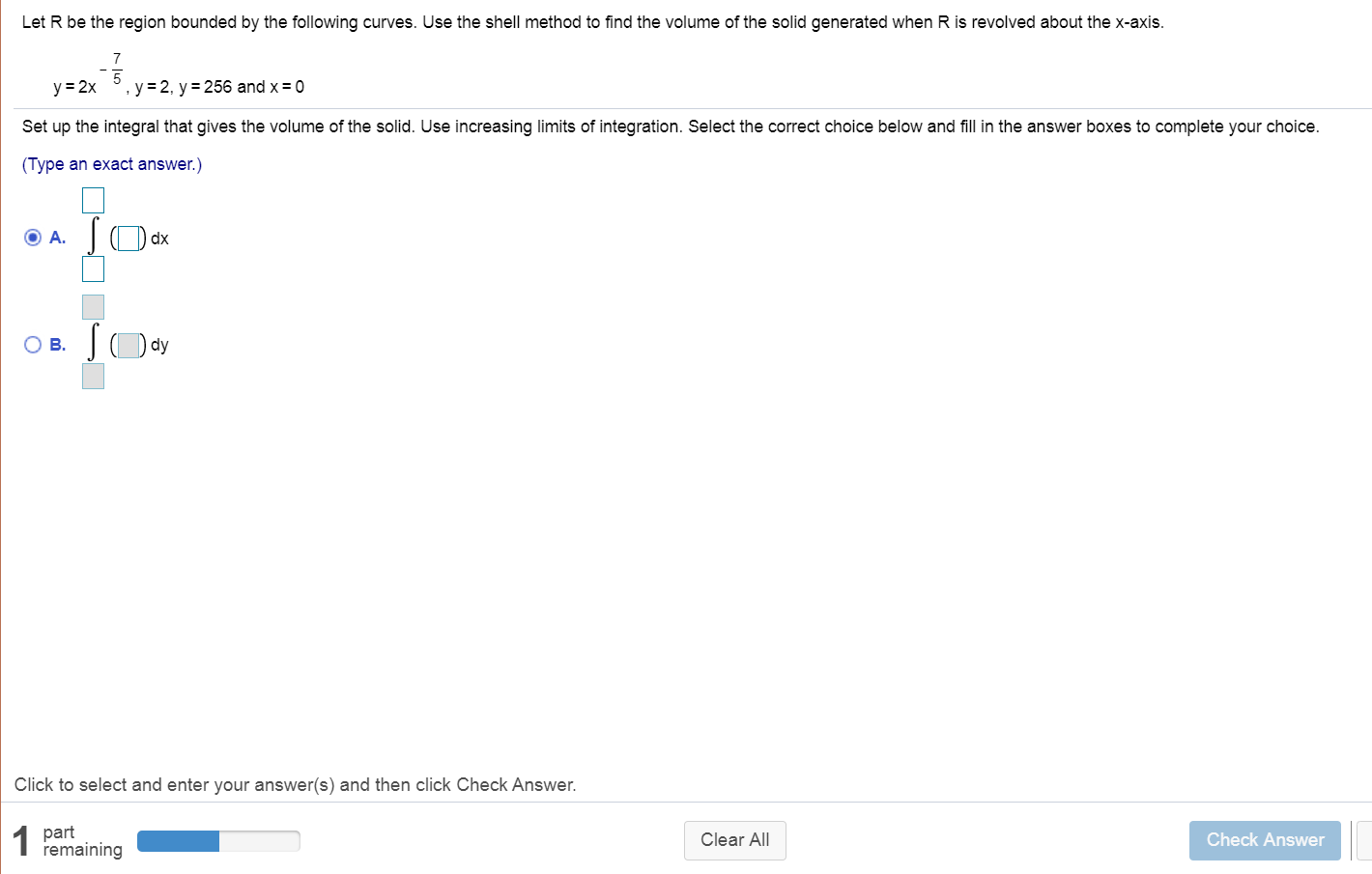# Let R be the region bounded by the following curves. Use the shell method to find the volume of the solid generated when R is revolved about the x-axis., y = 2, y = 256 and x = 0y = 2xSet up the integral that gives the volume of the solid. Use increasing limits of integration. Select the correct choice below and fill in the answer boxes to complete your choice.(Type an exact answer.)O A.B.Click to select and enter your answer(s) and then click Check Answer.part1 FemainingClear AllCheck Answer

Question
2 viewshelp_outlineImage TranscriptioncloseLet R be the region bounded by the following curves. Use the shell method to find the volume of the solid generated when R is revolved about the x-axis. , y = 2, y = 256 and x = 0 y = 2x Set up the integral that gives the volume of the solid. Use increasing limits of integration. Select the correct choice below and fill in the answer boxes to complete your choice. (Type an exact answer.) O A. B. Click to select and enter your answer(s) and then click Check Answer. part 1 Femaining Clear All Check Answer fullscreen
check_circle

Step 1

Consider the given functions:

Step 2

Draw the bounded region ...

### Want to see the full answer?

See Solution

#### Want to see this answer and more?

Solutions are written by subject experts who are available 24/7. Questions are typically answered within 1 hour.*

See Solution
*Response times may vary by subject and question.
Tagged in

### Calculus